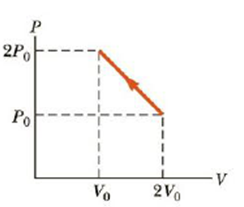Chapter 12, Problem 20P

Chapter
Section
Textbook Problem

A monatomic ideal gas under-goes the thermodynamic process shown in the PV diagram of Figure P12.20. Determine whether each of the values ΔU, Q, and W for the gas is positive, negative, or zero. Hint: The internal energy of a monatomic ideal gas at pressure P and occupying volume V is given by U = 3 2 PV.Figure P12.20

(a)

To determine
the work done on the gas.

Explanation

Given Info:

Initial volume of the gas is 2V0

Final volume of the gas is V0

Initial pressure of the gas is P0

Finial pressure of the gas is 2P0

The PV diagram of the gas is,

The area under the path on a PV diagram is equal to the magnitude of the work done on the gas.

Formula to calculate the area under PV diagram is,

A=(W1×H1)+12(B1×H2)

• W1 is the width of the rectangle A.
• H1 is the height of the rectangle A.
• B1 is the base of triangle B.
• H2 is the height of triangle B

(b)

To determine
To determine the change in internal energy of the ideal gas.

(c)

To determine
The energy (Q) in the process.

Still sussing out bartleby?

Check out a sample textbook solution.

See a sample solution

The Solution to Your Study Problems

Bartleby provides explanations to thousands of textbook problems written by our experts, many with advanced degrees!

Get Started

What does the word apparent mean in apparent visual magnitude?

Horizons: Exploring the Universe (MindTap Course List)

What are sister chromatids?

Human Heredity: Principles and Issues (MindTap Course List)

Where would you look for water in our solar system?

Oceanography: An Invitation To Marine Science, Loose-leaf Versin